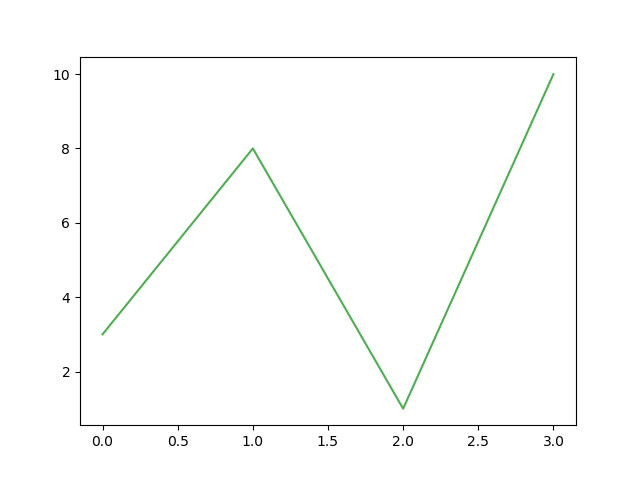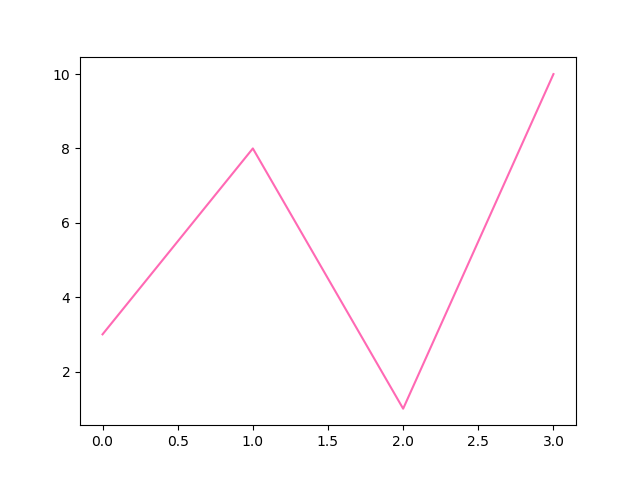## Python Tutorial

Python HOME Python Intro Python Get Started Python Syntax Python Comments Python Variables Python Data Types Python Numbers Python Casting Python Strings Python Booleans Python Operators Python Lists Python Tuples Python Sets Python Dictionaries Python If...Else Python While Loops Python For Loops Python Functions Python Lambda Python Arrays Python Classes/Objects Python Inheritance Python Iterators Python Polymorphism Python Scope Python Modules Python Dates Python Math Python JSON Python RegEx Python PIP Python Try...Except Python User Input Python String Formatting

## File Handling

Python File Handling Python Read Files Python Write/Create Files Python Delete Files

## Python Modules

NumPy Tutorial Pandas Tutorial SciPy Tutorial Django Tutorial

## Python Matplotlib

Matplotlib Intro Matplotlib Get Started Matplotlib Pyplot Matplotlib Plotting Matplotlib Markers Matplotlib Line Matplotlib Labels Matplotlib Grid Matplotlib Subplot Matplotlib Scatter Matplotlib Bars Matplotlib Histograms Matplotlib Pie Charts

## Machine Learning

Getting Started Mean Median Mode Standard Deviation Percentile Data Distribution Normal Data Distribution Scatter Plot Linear Regression Polynomial Regression Multiple Regression Scale Train/Test Decision Tree Confusion Matrix Hierarchical Clustering Logistic Regression Grid Search Categorical Data K-means Bootstrap Aggregation Cross Validation AUC - ROC Curve K-nearest neighbors

## Python MySQL

MySQL Get Started MySQL Create Database MySQL Create Table MySQL Insert MySQL Select MySQL Where MySQL Order By MySQL Delete MySQL Drop Table MySQL Update MySQL Limit MySQL Join

## Python MongoDB

MongoDB Get Started MongoDB Create Database MongoDB Create Collection MongoDB Insert MongoDB Find MongoDB Query MongoDB Sort MongoDB Delete MongoDB Drop Collection MongoDB Update MongoDB Limit

## Python Reference

Python Overview Python Built-in Functions Python String Methods Python List Methods Python Dictionary Methods Python Tuple Methods Python Set Methods Python File Methods Python Keywords Python Exceptions Python Glossary

## Module Reference

Random Module Requests Module Statistics Module Math Module cMath Module

## Python How To

Remove List Duplicates Reverse a String Add Two Numbers

## Python Examples

Python Examples Python Compiler Python Exercises Python Quiz Python Bootcamp Python Certificate

# Matplotlib Line

## Linestyle

You can use the keyword argument `linestyle`, or shorter `ls`, to change the style of the plotted line:

### Example

Use a dotted line:

import matplotlib.pyplot as plt
import numpy as np

ypoints = np.array([3, 8, 1, 10])

plt.plot(ypoints, linestyle = 'dotted')
plt.show()

### Result:Try it Yourself »

### Example

Use a dashed line:

plt.plot(ypoints, linestyle = 'dashed')

### Result:Try it Yourself »

## Shorter Syntax

The line style can be written in a shorter syntax:

`linestyle` can be written as `ls`.

`dotted` can be written as `:`.

`dashed` can be written as `--`.

### Example

Shorter syntax:

plt.plot(ypoints, ls = ':')

### Result:Try it Yourself »

## Line Styles

You can choose any of these styles:

Style Or
'solid' (default) '-' Try it »
'dotted' ':' Try it »
'dashed' '--' Try it »
'dashdot' '-.' Try it »
'None' '' or ' ' Try it »

## Line Color

You can use the keyword argument `color` or the shorter `c` to set the color of the line:

### Example

Set the line color to red:

import matplotlib.pyplot as plt
import numpy as np

ypoints = np.array([3, 8, 1, 10])

plt.plot(ypoints, color = 'r')
plt.show()

### Result:Try it Yourself »

You can also use Hexadecimal color values:

### Example

Plot with a beautiful green line:

...
plt.plot(ypoints, c = '#4CAF50')
...

### Result:Try it Yourself »

Or any of the 140 supported color names.

### Example

Plot with the color named "hotpink":

...
plt.plot(ypoints, c = 'hotpink')
...

### Result:Try it Yourself »

## Line Width

You can use the keyword argument `linewidth` or the shorter `lw` to change the width of the line.

The value is a floating number, in points:

### Example

Plot with a 20.5pt wide line:

import matplotlib.pyplot as plt
import numpy as np

ypoints = np.array([3, 8, 1, 10])

plt.plot(ypoints, linewidth = '20.5')
plt.show()

### Result:Try it Yourself »

## Multiple Lines

You can plot as many lines as you like by simply adding more `plt.plot()` functions:

### Example

Draw two lines by specifying a `plt.plot()` function for each line:

import matplotlib.pyplot as plt
import numpy as np

y1 = np.array([3, 8, 1, 10])
y2 = np.array([6, 2, 7, 11])

plt.plot(y1)
plt.plot(y2)

plt.show()

### Result:Try it Yourself »

You can also plot many lines by adding the points for the x- and y-axis for each line in the same `plt.plot()` function.

(In the examples above we only specified the points on the y-axis, meaning that the points on the x-axis got the the default values (0, 1, 2, 3).)

The x- and y- values come in pairs:

### Example

Draw two lines by specifiyng the x- and y-point values for both lines:

import matplotlib.pyplot as plt
import numpy as np

x1 = np.array([0, 1, 2, 3])
y1 = np.array([3, 8, 1, 10])
x2 = np.array([0, 1, 2, 3])
y2 = np.array([6, 2, 7, 11])

plt.plot(x1, y1, x2, y2)
plt.show()

### Result:Try it Yourself »